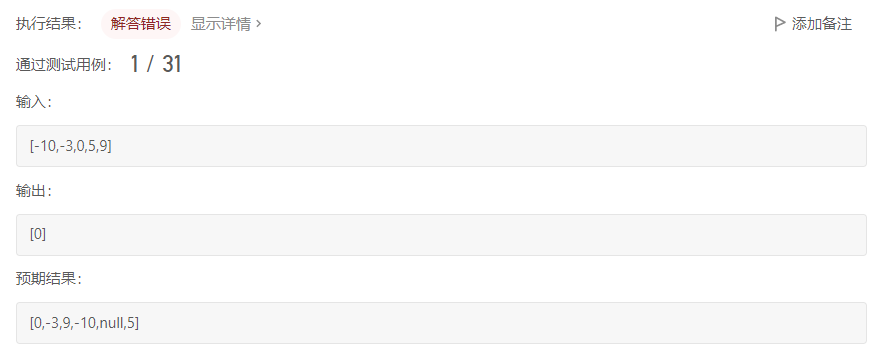# 将所有序数组转换为二叉搜索树

``````/**
* Definition for a binary tree node.
* struct TreeNode {
*     int val;
*     struct TreeNode *left;
*     struct TreeNode *right;
* };
*/

struct TreeNode* lr(int *nums, int l, int r, struct TreeNode* rt)
{
if(l>r)
return NULL;
rt=(struct TreeNode*)malloc(sizeof(struct TreeNode));
rt->left=rt->right=NULL;
int mid=l + (r-l)/2;
rt->val=nums[mid];
lr(nums,l,mid-1,rt->left);
lr(nums,mid+1,r,rt->right);
return rt;
}

struct TreeNode* sortedArrayToBST(int* nums, int numsSize){
struct TreeNode *root;
root=lr(nums,0,numsSize-1,root);
return root;
}

``````• 写回答

#### 3条回答默认 最新

•再生产中 2023-02-09 13:18
关注

这题涉及到的主要问题就是传参，应当使用二级指针作为实参传递，通过的代码如下：

``````

/**
* Definition for a binary tree node.
* struct TreeNode {
*     int val;
*     struct TreeNode *left;
*     struct TreeNode *right;
* };
*/

struct TreeNode* lr(int *nums, int l, int r, struct TreeNode** rt)
{
if(l>r)
return NULL;
struct TreeNode* rtt=(struct TreeNode*)malloc(sizeof(struct TreeNode));
rtt->left=rtt->right=NULL;
int mid=l + (r-l)/2;
rtt->val=nums[mid];
*rt=rtt;
lr(nums,l,mid-1,&(rtt->left));
lr(nums,mid+1,r,&(rtt->right));
return rtt;
}

struct TreeNode* sortedArrayToBST(int* nums, int numsSize){
struct TreeNode *root;
root=lr(nums,0,numsSize-1,&root);
return root;
}

``````

关于传参问题毕竟清晰易懂的解释可以参考这个https://blog.csdn.net/Kukeoo/article/details/114297192?spm=1001.2014.3001.5506

本回答被题主选为最佳回答 , 对您是否有帮助呢?
评论

#### 悬赏问题

• ¥20 powerbulider 导入excel文件，显示不完整
• ¥20 #关于multisim绘图遇到的问题
• ¥15 用keil调试程序保证结果进行led相关闪烁